# Add Marginal Histograms to 'ggplot2', and More 'ggplot2' Enhancements

Collection of functions and layers to enhance 'ggplot2'. The flagship function is 'ggMarginal()', which can be used to add marginal histograms/boxplots/density plots to 'ggplot2' scatterplots.

# ggExtra - Add marginal histograms to ggplot2, and more ggplot2 enhancements

the MIT license.*

`ggExtra` is a collection of functions and layers to enhance ggplot2. The flagship function is `ggMarginal`, which can be used to add marginal histograms/boxplots/density plots to ggplot2 scatterplots. You can view a live interactive demo to test it out!

Most other functions/layers are quite simple but are useful because they are fairly common ggplot2 operations that are a bit verbose.

This is an instructional document, but I also wrote a blog post about the reasoning behind and development of this package.

Note: it was brought to my attention that several years ago there was a different package called `ggExtra`, by Baptiste (the author of `gridExtra`). That old `ggExtra` package was deleted in 2011 (two years before I even knew what R is!), and this package has nothing to do with the old one.

## Installation

`ggExtra` is available through both CRAN and GitHub.

To install the CRAN version:

``````install.packages("ggExtra")
``````

To install the latest development version from GitHub:

``````install.packages("devtools")
devtools::install_github("daattali/ggExtra")
``````

## Marginal plots RStudio addin/gadget

`ggExtra` comes with an addin for `ggMarginal()`, which lets you interactively add marginal plots to a scatter plot. To use it, simply highlight the code for a ggplot2 plot in your script, and select ggplot2 Marginal Plots from the RStudio Addins menu. Alternatively, you can call the addin directly by calling `ggMarginalGadget(plot)` with a ggplot2 plot.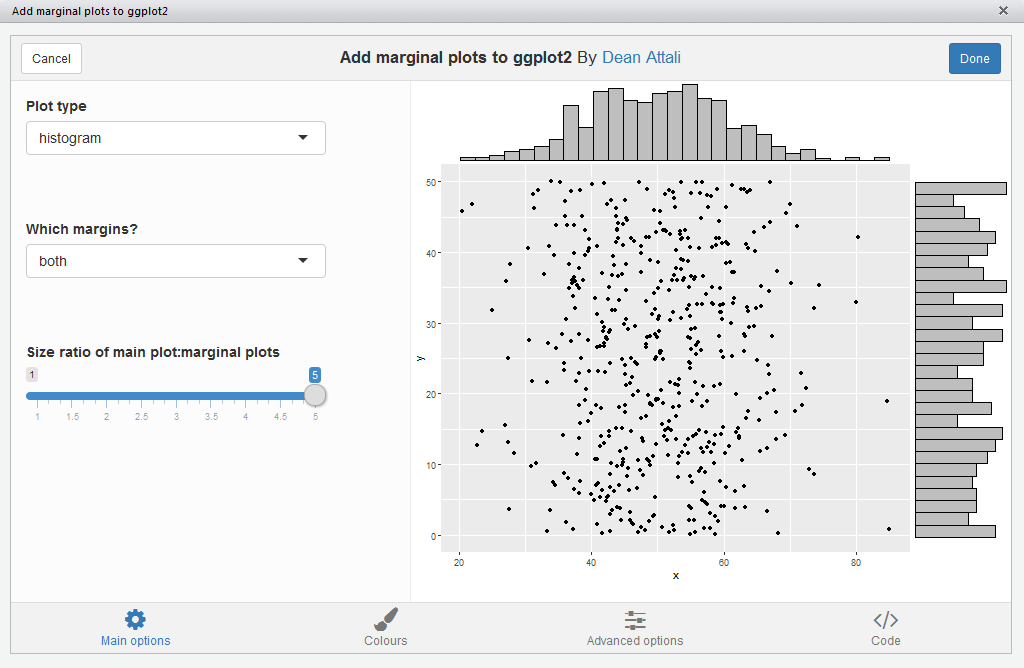## Usage

We’ll first load the package and ggplot2, and then see how all the functions work.

``````library("ggExtra")
library("ggplot2")
``````

## `ggMarginal` - Add marginal histograms/boxplots/density plots to ggplot2 scatterplots

`ggMarginal()` is an easy drop-in solution for adding marginal density plots/histograms/boxplots to a ggplot2 scatterplot. The easiest way to use it is by simply passing it a ggplot2 scatter plot, and `ggMarginal()` will add the marginal plots.

As a simple first example, let’s create a dataset with 500 points where the x values are normally distributed and the y values are uniformly distributed, and plot a simple ggplot2 scatterplot.

``````set.seed(30)
df1 <- data.frame(x = rnorm(500, 50, 10), y = runif(500, 0, 50))
p1 <- ggplot(df1, aes(x, y)) + geom_point() + theme_bw()
p1
``````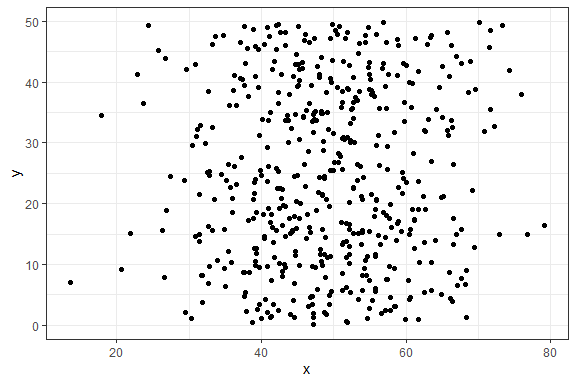And now to add marginal density plots:

``````ggMarginal(p1)
``````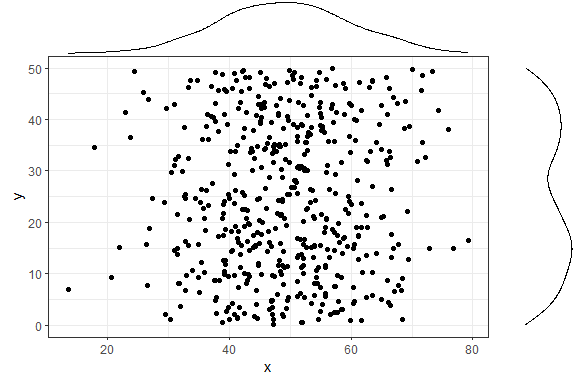That was easy. Notice how the syntax does not follow the standard ggplot2 syntax - you don’t “add” a ggMarginal layer with `p1 + ggMarginal()`, but rather ggMarginal takes the object as an argument and returns a different object. This means that you can use magrittr pipes, for example `p1 %>% ggMarginal()`.

Let’s make the text a bit larger to make it easier to see.

``````ggMarginal(p1 + theme_bw(30) + ylab("Two\nlines"))
``````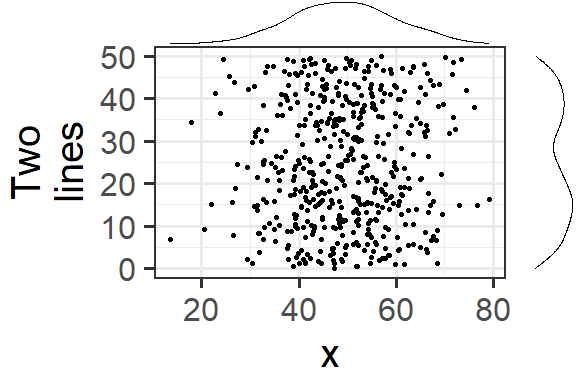Notice how the marginal plots occupy the correct space; even when the main plot’s points are pushed to the right because of larger text or longer axis labels, the marginal plots automatically adjust.

If your scatterplot has a factor variable mapping to a colour (ie. points in the scatterplot are colour-coded according to a variable in the data, by using `aes(colour = ...)`), then you can use `groupColour = TRUE` and/or `groupFill = TRUE` to reflect these groupings in the marginal plots. The result is multiple marginal plots, one for each colour group of points. Here’s an example using the iris dataset.

``````piris <- ggplot(iris, aes(Sepal.Length, Sepal.Width, colour = Species)) +
geom_point()
ggMarginal(piris, groupColour = TRUE, groupFill = TRUE)
``````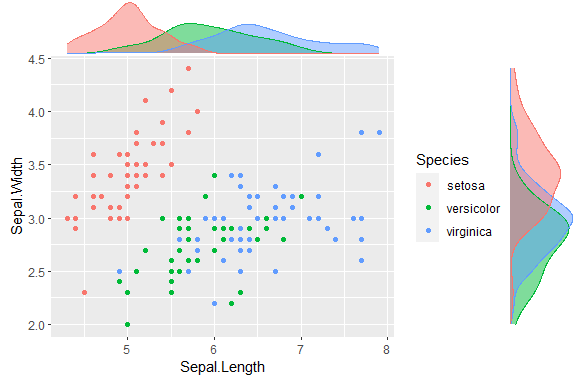You can also show histograms instead.

``````ggMarginal(p1, type = "histogram")
``````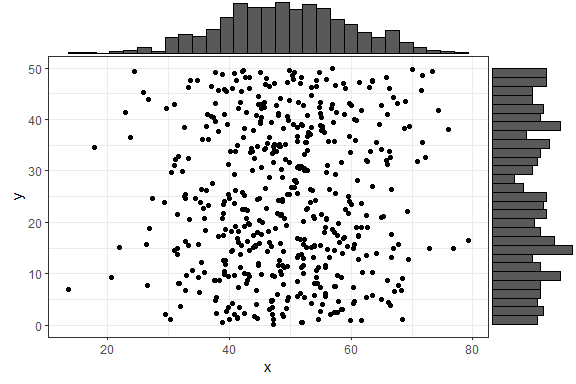There are several more parameters, here is an example with a few more being used. Note that you can use any parameters that the `geom_XXX()` layers accept, such as `col` and `fill`, and they will be passed to these layers.

``````ggMarginal(p1, margins = "x", size = 2, type = "histogram",
col = "blue", fill = "orange")
``````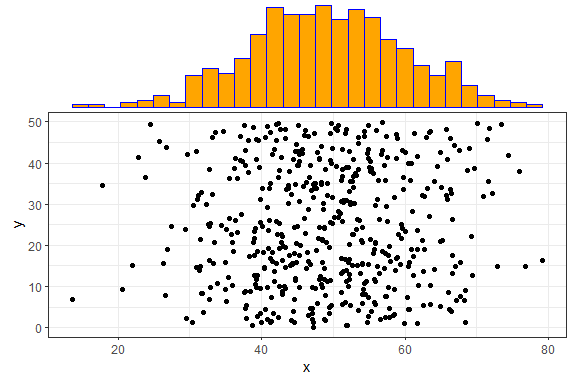In the above example, `size = 2` means that the main scatterplot should occupy twice as much height/width as the margin plots (default is 5). The `col` and `fill` parameters are simply passed to the ggplot layer for both margin plots.

If you want to specify some parameter for only one of the marginal plots, you can use the `xparams` or `yparams` parameters, like this:

``````ggMarginal(p1, type = "histogram", xparams = list(binwidth = 1, fill = "orange"))
``````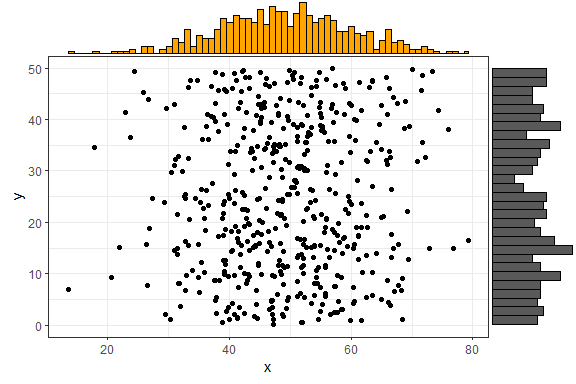You don’t have to supply a ggplot2 scatterplot, you can also just tell ggMarginal what dataset and variables to use, but of course this way you lose the ability to customize the main plot (change text/font/theme/etc).

``````ggMarginal(data = mtcars, x = "wt", y = "mpg")
``````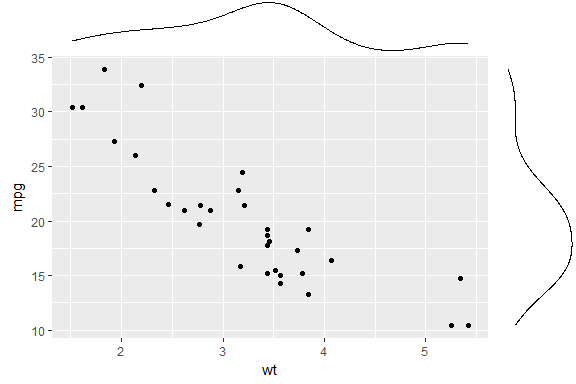Last but not least - you can also save the output from `ggMarginal` and display it later. (This may sound trivial, but it was not an easy problem to solve - see this discussion).

``````p <- ggMarginal(p1)
p
``````You can also create marginal box plots and violin plots. For more information, see `?ggExtra::ggMarginal`.

## `removeGrid` - Remove grid lines from ggplot2

This is just a convenience function to save a bit of typing and memorization. Minor grid lines are always removed, and the major x or y grid lines can be removed as well (default is to remove both).

`removeGridX` is a shortcut for `removeGrid(x = TRUE, y = FALSE)`, and `removeGridY` is similarly a shortcut for… .

``````df2 <- data.frame(x = 1:50, y = 1:50)
p2 <- ggplot2::ggplot(df2, ggplot2::aes(x, y)) + ggplot2::geom_point()
p2 + removeGrid()
``````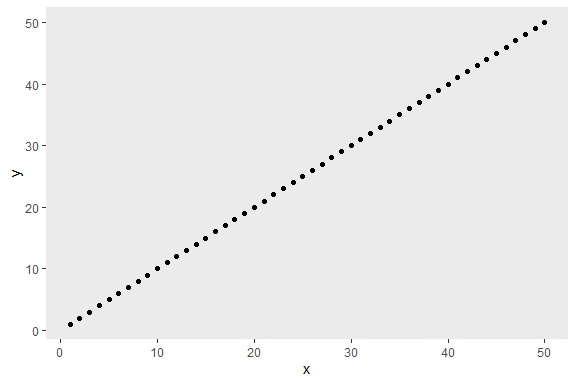For more information, see `?ggExtra::removeGrid`.

## `rotateTextX` - Rotate x axis labels

Often times it is useful to rotate the x axis labels to be vertical if there are too many labels and they overlap. This function accomplishes that and ensures the labels are horizontally centered relative to the tick line.

``````df3 <- data.frame(x = paste("Letter", LETTERS, sep = "_"),
y = seq_along(LETTERS))
p3 <- ggplot2::ggplot(df3, ggplot2::aes(x, y)) + ggplot2::geom_point()
p3 + rotateTextX()
``````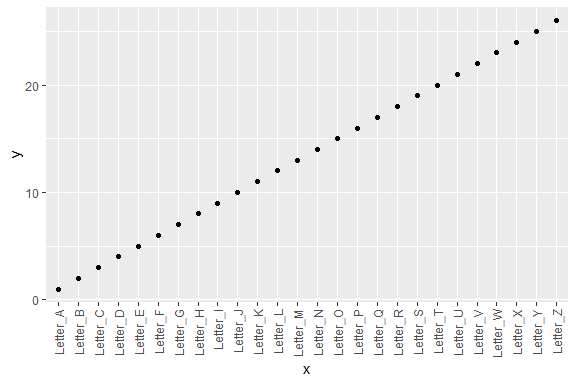For more information, see `?ggExtra::rotateTextX`.

## `plotCount` - Plot count data with ggplot2

This is a convenience function to quickly plot a bar plot of count (frequency) data. The input must be either a frequency table (obtained with `base::table`) or a data.frame with 2 columns where the first column contains the values and the second column contains the counts.

An example using a table:

``````plotCount(table(infert\$education))
``````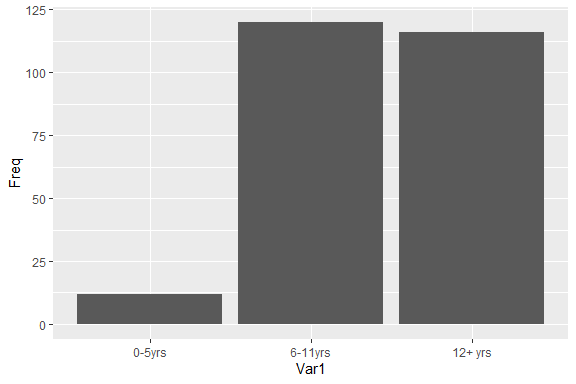An example using a data.frame:

``````df4 <- data.frame("vehicle" = c("bicycle", "car", "unicycle", "Boeing747"),
"NumWheels" = c(2, 4, 1, 16))
plotCount(df4) + removeGridX()
``````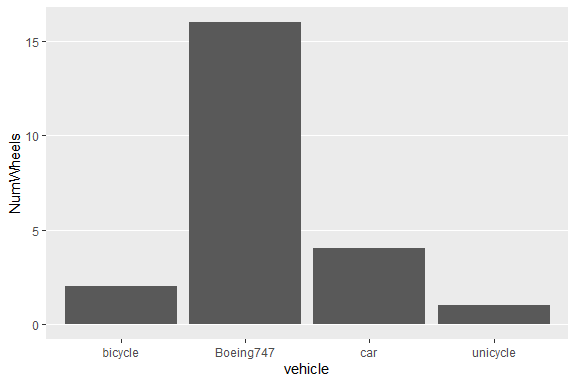For more information, see `?ggExtra::plotCount`.

# ggExtra 0.8

NEW FEATURES

• Added support for violin plots (#62)
• Added support for mapping colour from the scatter plot to colour/fill in the marginal plots (#61)

BUX FIXES

• Make sure marginal data comes from correct data frame (#67)
• Fix #81: many issues when the x or y axis have custom scales applied (#101)
• Fix #99: plot subtitle was in the wrong position when no title was used (#103)

# ggExtra 0.7

2017-06-21

### Refactoring of `ggMarginal()`:

• Several of the stages that `ggMarginal` completes to create the final plot are now broken into (more) helper functions
• Code that uses internal ggplot2 structure works on different versions of ggplot2
• Removed code that added y labels to marginal plot to deal with long y labels as well as spacing issues (https://github.com/daattali/ggExtra/blob/6c4923c2ad2e700226cdcdc9666de513d6ae3a41/R/ggMarginal.R#L186-L201)
• Removed code that set the marginal plot limits when the marginal plot was a boxplot (https://github.com/daattali/ggExtra/blob/6c4923c2ad2e700226cdcdc9666de513d6ae3a41/R/ggMarginalHelpers.R#L177)

### Other features

• add visual tests
• add tests over different versions of ggplot2, to ensure backwards compatibility
• add arguments to `rotateTextX()`

# ggExtra 0.6

2016-11-11

• support new ggplot2 v2.2.0 (not backwards compatible unforunately because ggplot2 internals changed too much)

# ggExtra 0.5.2

2016-10-03

• use `colourpicker` package instead of deprecated colour input from shinyjs
• bug fix: retain the title font face (#30)

# ggExtra 0.5.1

2016-07-25

• UI improvements to shiny app
• add social media meta tags to shiny app

# ggExtra 0.5

2016-05-29

• ggMarginal now supports plots with legends (thanks to @crew102) (#23)

# ggExtra 0.4.2

2016-04-30

• ggMarginal addin now works on all screen resolutions (#24)

# ggExtra 0.4.1

2016-04-24

• Remove hack required by old version of `gridExtra`

# ggExtra 0.4.0

2016-03-25

• Added an RStudio addin and gadget for creating ggplot2 marginal plots (select ggplot2 Marginal Plots from the RStudio Addins menu, or call `ggExtra::ggMarginalGadget(plot)`)

# ggExtra 0.3.3

2015-12-14

• Small UI changes to the Shiny app demo

# ggExtra 0.3.2

2015-11-10

• Fixed bug where using `ggplot2::set_theme()` was causing the marginal plots to also use that theme

# ggExtra 0.3.1

2015-11-05

• Fixed bug where first and last bins of histograms were never showing (#18)
• Finally tackled a long standing problem: if main plot has a title, move the title on top of the marginal plots. An unwanted side effect of this is that the title font size will not be retained because the title is its own grob. (#3)

# ggExtra 0.3.0

2015-09-02

• significant internal refactoring of `ggMarginal` to make it work with new ggplot2 version (after version 1.0.1 ggplot2 had tons of breaking changes) (some parts of the function use different code depending on the version of ggplot2 installed, I hope this doesn't raise any bugs)
• make `ggMarginal` a little more robust to many different theme options so that even if the main plot changes the tick mark lengths or x axis size or many different options, the marginal plots will still align properly
• add more usage examples to `ggMarginal`

# ggExtra 0.2.3

2015-08-19

• bug fix: ggMarginal now works when the original plot has expressions as the x/y variables. For example, calling ggMarginal on a plot that had `aes(x+10, log(y))` did not work before

# ggExtra 0.2.2

2015-08-17

• simplify and remove some unneeded package checks since `grid` and `gridExtra` should be installed automatically

# ggExtra 0.2.1

2015-08-04

minor changes

• small updates to ggMarginal demo shiny app
• small changes to ggMarginal documentation
• small changes to package DESCRIPTION

# ggExtra 0.2.0

2015-07-10

• marginal plots now use the same axis transformations (log/reverse/limits/etc) as the main plot
• rewrote `ggMarginal` to support the new `gridExtra` package which has been completely rewritten after 2 years of inactivity

# ggExtra 0.1.6

2015-06-26

• added `...` parameter to `plotCount` after a request to add a way to colour the bars

# ggExtra 0.1.5

2015-06-08

• ggMarginal: add support for boxplots
• ggMarginal: add `...` parameter that allows you to pass any arguments to the corresponding ggplot2 geom layer
• ggMarginal: add `xparams` and `yparams` parameters to pass any arguments to only the x/y marginal plot
• BREAKING CHANGE: ggMarginal: `marginCol` and `marginFill` params have been removed since `colour` and `fill` can be provided as regular params thanks to the `...` parameter

# ggExtra 0.1.1

2015-04-21

Add a Shiny app that shows how to use `ggMarginal`, can be viewed with `runExample` or on my Shiny Server

# ggExtra 0.1.0

2015-03-28

Package is officially released to the public and is now on CRAN

# Reference manual

install.packages("ggExtra")

0.8 by Dean Attali, a year ago

https://github.com/daattali/ggExtra

Report a bug at https://github.com/daattali/ggExtra/issues

Browse source code at https://github.com/cran/ggExtra

Authors: Dean Attali [aut, cre] , Christopher Baker [aut]

Documentation:   PDF Manual

MIT + file LICENSE license

System requirements: pandoc with https support

Imported by SCVA, SHAPforxgboost, SHELF, bamdit, dextergui, ggstatsplot, jarbes.

Suggested by BlandAltmanLeh, DataVisualizations, Sofi, ddpcr, sensitivity.

See at CRAN